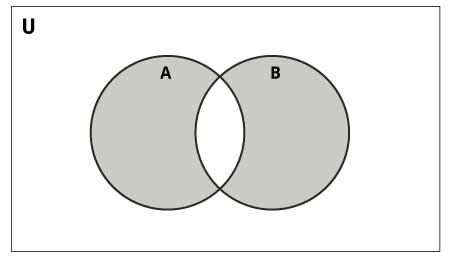# Python Set symmetric_difference()

The symmetric_difference() returns a new set which is the symmetric difference of two sets.

The symmetric difference of two sets A and B is the set of elements which are in either of the sets A or B but not in both.The syntax of symmetric_difference() is:

`A.symmetric_difference(B)`

## symmetric_difference() Parameters

The symmetric_difference takes a single argument (set).

## Return Value from symmetric_difference()

The symmetric_difference() returns a new set which is the symmetric difference of sets A and B.

## Example 1: How symmetric_difference() works in Python?

```A = {'a', 'b', 'c', 'd'}
B = {'c', 'd', 'e' }
C = {}

print(A.symmetric_difference(B))
print(B.symmetric_difference(A))

print(A.symmetric_difference(C))
print(B.symmetric_difference(C))
```

When you run the program, the output will be:

```{'b', 'a', 'e'}
{'b', 'e', 'a'}
{'b', 'd', 'c', 'a'}
{'d', 'e', 'c'}```

In Python, you can also find the symmetric difference using ^ operator.

```A = {'a', 'b', 'c', 'd'}
B = {'c', 'd', 'e' }

print(A ^ B)
print(B ^ A)

print(A ^ A)
print(B ^ B)
```

When you run the program, the output will be:

```{'e', 'a', 'b'}
{'e', 'a', 'b'}
set()
set()```